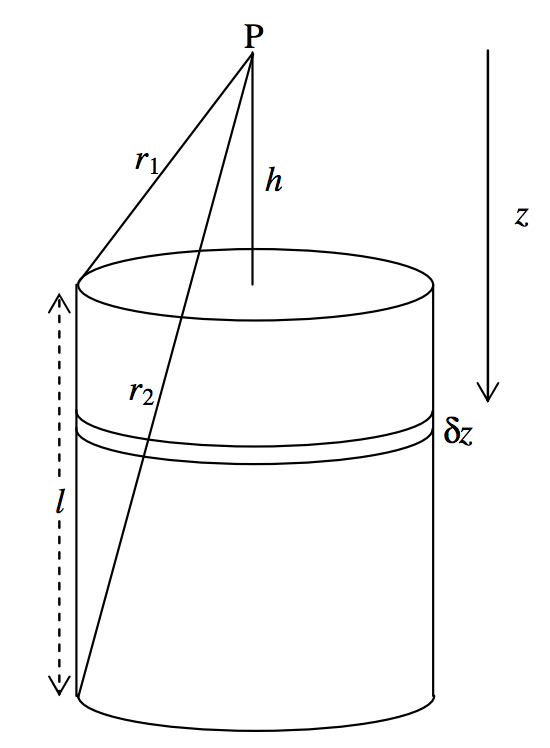$$\require{cancel}$$

# 5.4.7: Solid Cylinder

•• Contributed by Jeremy Tatum
• Emeritus Professor (Physics & Astronomy) at University of Victoria

We do this not because it has any particular relevance to celestial mechanics, but because it is easy to do. We imagine a solid cylinder, density $$ρ$$, radius $$a$$, length $$l$$. We seek to calculate the field at a point $$\text{P}$$ on the axis, at a distance $$h$$ from one end of the cylinder (figure $$\text{V.8}$$).$$\text{FIGURE V.8}$$

The field at $$\text{P}$$ from an elemental disc of thickness $$δz$$ a distance z below P is (from equation 5.4.9)

$δg = Gρδzω. \label{5.4.19} \tag{5.4.19}$

Here $$ω$$ is the solid angle subtended at $$\text{P}$$ by the disc, which is $$2 \pi \left[ 1 - \frac{z}{\left( z^2 + a^2 \right)^{1/2}} \right]$$. Thus the field at $$\text{P}$$ from the entire cylinder is

$g = 2 \pi G ρ \int_h^{l+h} \left[ 1 - \frac{z}{(z^2 + a^2)^{1/2}} \right] dz, \label{5.4.20} \tag{5.4.20}$

or $g = 2 \pi G ρ \left( l - \sqrt{(l+h)^2 + a^2} + \sqrt{h^2+ a^2} \right), \label{5.4.21} \tag{5.4.21}$

or $g = 2\pi G ρ ( l - r_2 + r_1 ). \label{5.4.22} \tag{5.4.22}$

It might also be of interest to express $$g$$ in terms of the height $$y (= \frac{1}{2}l + h)$$ of the point $$\text{P}$$ above the mid-point of the cylinder. Instead of equation $$\ref{5.4.21}$$, we then have

$g = 2 \pi G ρ \left( l - \sqrt{(y + \frac{1}{2}l)^2 + a^2} + \sqrt{(y - \frac{1}{2}l)^2 + a^2} \right) . \label{5.4.23} \tag{5.4.23}$

If the point $$\text{P}$$ is inside the cylinder,at a distance $$h$$ below the upper end of the cylinder, the limits of integration in equation $$\ref{5.4.20}$$ are $$h$$ and $$l − h$$ , and the distance $$y$$ is $$\frac{1}{2}l − h$$. In terms of $$y$$ the gravitational field at $$\text{P}$$ is then

$g = 2 \pi G ρ \left( 2y - \sqrt{(y + \frac{1}{2}l)^2 + a^2} + \sqrt{(y-\frac{1}{2}l)^2 + a^2} \right). \label{5.4.24} \tag{5.4.24}$

In the graph below I have assumed, by way of example, that $$l$$ and $$a$$ are both 1, and I have plotted $$g$$ in units of $$2 \pi Gρ$$ (counting $$g$$ as positive when it is directed downwards) from $$y = −1$$ to $$y = + 1$$. The portion inside the cylinder $$(- \frac{1}{2} ≤ y ≤ \frac{1}{2}l)$$, represented by equation $$\ref{5.4.24}$$, is almost, but not quite, linear. The field at the centre of the cylinder is, of course, zero.Below, I draw the same graph, but for a thin disc, with $$a = 1$$ and $$l = 0.1$$. We see how it is that the field reaches a maximum immediately above or below the disc, but is zero at the centre of the disc.